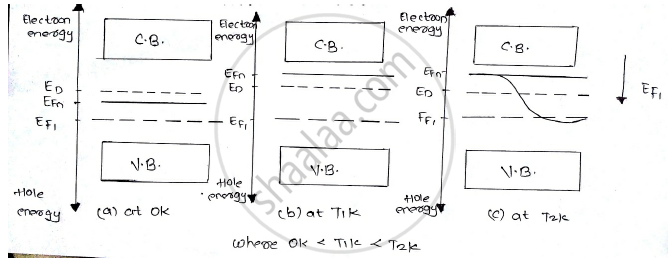BE Biomedical Engineering Semester 1 (FE First Year)University of Mumbai
Share
Notifications

View all notifications
Books Shortlist
Your shortlist is empty

# Write Fermi Dirac distribution function. With the help of diagram. Explain the variation of Fermi level with temperature in n-type semiconductor. - BE Biomedical Engineering Semester 1 (FE First Year) - Applied Physics 1

Login
Create free account

Forgot password?
ConceptFermi Dirac Distribution Function

#### Question

Write Fermi Dirac distribution function. With the help of diagram. Explain the variation of Fermi level with temperature in n-type semiconductor.

#### Solution

Each energy band in a crystal accommodates a large number of electron energy levels. According to Pauli’s exclusion principle any energy level can be occupied by two electrons only, one spin up and down . however, all the available energy states are not filled in an energy band.
The separation between the consecutive energy level is very small around 10-27 eV due to which the energy states are not filled in an energy band.

FERMI DIRAC DISTRIBUTION FUNCTION.
The carrier occupancy of the energy states is represented by a continuous distribution function known as the Fermi-Dirac distribution function, given by

f(E) = 1/(1+e^((E-E_F)/(kT))

This indicates the probability that a particular quantum state at the energy level E is occupied by an electron. Here k is Boltzmann’s constant and T is absolute temperature of the semiconductor. The energy EF called Fermi energy that corresponds to a reference level  called Fermi level.

IN n-TYPE SEMICONDUCTOR.
⦁ At 0K the fermi level EFn lies between the conduction band and the donor level.

⦁ As temperature increases more and more electrons shift to the conduction band leaving behind equal number of holes in the valence band. These electron hole pairs are intrinsic carriers.
⦁ With the increase in temperature the intrinsic carriers dominate the donors.

⦁ To maintain the balance of the carrier density on both sides the fermi level EFn gradually shifts downwards.
⦁ Finally at high temperature when the donor density is almost negligible EFn is very close to EFn .Is there an error in this question or solution?

#### APPEARS IN

Solution Write Fermi Dirac distribution function. With the help of diagram. Explain the variation of Fermi level with temperature in n-type semiconductor. Concept: Fermi Dirac Distribution Function.
S